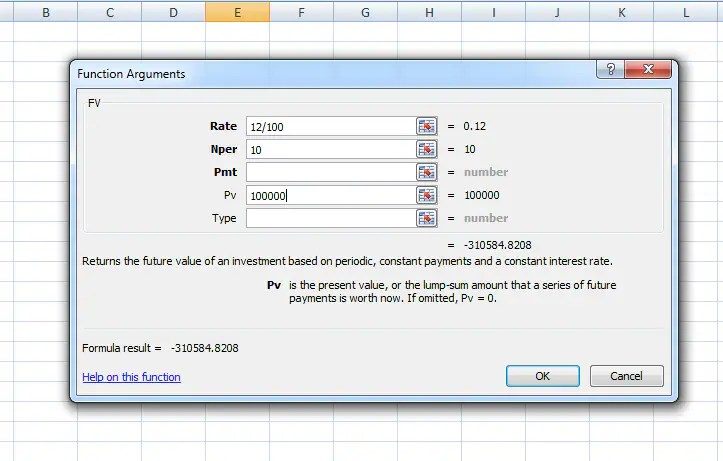Home > Finance > How much will your investments be worth after 10 years

# How much will your investments be worth after 10 years

101 ViewsThe most common question an investor asks is how much will his investments grow in next 10 years or 15 years or any other time period, or, what will be the worth of his investments after 10 years an so on. Well, its an easy calculation. You don’t need to depend on an expert or your investment planner for this simple calculation. You can either use an excel sheet or if you are not comfortable working on an excel sheet, use a pen and a paper to calculate. We will discuss both the methods here:

How to calculate the value of your investments after 10 years, using an excel sheet

There can be two cases:

-Lumpsum investment

-Regular investment on a monthly basis (just like, monthly SIP in a mutual fund)

Lumpsum Investment

Future value of your lumpsum investment can be calculated using ‘Insert Function’ under the Formula tab on an excel sheet. Then choose ‘FV’ of Future Value option. Suppose, you want to calculate the future value of 1 lakh invested at 12% rate of return, after 10 years, here’s howto enter the figures in excel formula:

The value of 1 lakh invested today at 12% for 10 years will become 3,10,585. Here, if you are not investing in a fixed return instrument, you have to assume the rate of return that you believe your investment will earn.

This can also help you to find the future value of your goal. Let’s put it like this: how much will you spend on a computer course that costs 1 lakh today, after 10 years assuming the education inflation is 12% per year.

Regular investment on a monthly basis (just like, monthly SIP in a mutual fund)

Just like we do SIP in a mutual fund, if you invest 10,000 every month for eight years, assuming 12% annual returns, here’s how to enter these values in the same ‘FV’ formula. Since its monthly investment, the rate of return has to be divided by 12 and tenure or number of years have to be multiplied by 12.Future value calculation of a series (like SIP in mutual fund) of investment in excel

The future value of the monthly investment would be close to 16 lakh.

How to calculate the future value of your investment, using a paper and a pen

Not an excel user, no worries, you can try the formulas given below and calculate your investment’s future value.

Lumpsum investment

The formula is :

FV= PV x (1+r%)^n

Using the above mentioned illustration,

FV= Future value,

PV= present value of your investment = 1,00,000

r= rate of return =12

n= time period of investment = 10 years

Putting the values in the formula,

FV=1,00,000 x ((1+0.12)^10)

FV= 3,10,585

Regular investment on a monthly basis (just like, monthly SIP in a mutual fund)

Here’s the formula:

FV= I x (((1+r%)^n) -1)/r%)

Where,

FV=Future value,

I=Monthly investment = 10,000

r= rate of return = 12/12 = 1( divide the interest rate by 12 since ite monthly investment)

n= time period of investment = 8 years x 12 months=96

Entering the value in the formula,

FV= 10,000 x (((1+0.01)^96)-1)/0.01

FV= 15,99,273 or 16 lakh

These formulas can also be used to find out the future value of your current expenses or goals. And once you have an idea about future figures, you can plan your investments accordingly.

Subscribe to newsletters

* Enter a valid email

* Thank you for subscribing to our newsletter.

Source linkHi guys, this is Kimmy, I started LicensetoBlog to help you with the latest updated news about the world with daily updates from all leading news sources. Beside, I love to write about several niches like health, business, finance, travel, automation, parenting and about other useful topics to keep you find the the original information on any particular topic. Hope you will find LicensetoBlog helpful in various ways. Keep blogging and help us grow as a community for internet lovers.

Related Articles## Gold prices fall sharply. Should you start buy...## Milkha Singh: An unmatchable romance with a ne...## SUVs rule the roads with 48.5% market share in...